# Volume 29, 2023, Number 1

Volume 29 ▶ Number 1 ▷ Number 2Number 3Number 4 (Online First)

Pauli–Leonardo quaternions
Original research paper. Pages 1—16
Zehra İşbilir, Mahmut Akyiğit and Murat Tosun

In this study, we define Pauli–Leonardo quaternions by taking the coefficients of the Pauli quaternions as Leonardo numbers. We give the recurrence relation, Binet formula, generating function, exponential generating function, some special equalities, and the sum properties of these novel quaternions. In addition, we investigate the interrelations between Pauli–Leonardo quaternions and the Pauli–Fibonacci, Pauli–Lucas quaternions. Moreover, we create some algorithms that determine the terms of the Pauli–Leonardo quaternions. Finally, we generate the matrix representations of the Pauli–Leonardo quaternions and ℝ-linear transformations.

On distribution of the number of semisimple rings of order at most x in an arithmetic progression
Original research paper. Pages 17—23
Thorranin Thansri, Teerapat Srichan and Pinthira Tangsupphathawat

Let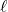and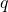denote relatively prime positive integers. In this article, we derive the asymptotic formula for the summation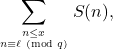where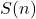denotes the number of non-isomorphic finite semisimple rings with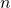elements.

A note on the number an+ bndcn
Original research paper. Pages 24—29
Nguyen Viet Dung and Luu Ba Thang

We say that a positive integer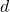is special number of degreeif for every integer, there exist nonzero integerssuch that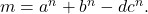In this paper, we investigate some necessary conditions onfor existing a special number of degree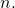Two generalizations of Liouville λ function
Original research paper. Pages 30—39
André Pierro de Camargo

We study the properties of two classes of functions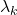and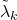that generalize the Liouville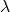function, including some equivalencies between the Riemann hypothesis and some assertions about the asymptotic behavior of the summatory functions ofand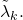Similar results are obtained for the generalization of the Möbius function considered by Tanaka.

A study of the complexification process of the (s,t)-Perrin sequence
Original research paper. Pages 40—47
Renata Passos Machado Vieira, Francisco Regis Vieira Alves and Paula Maria Machado Cruz Catarino

The present article deals with the study of the generalized (s,t)-Perrin sequence in its complex process. Thus, from the one-dimensional model of the generalized (s,t)-Perrin sequence, imaginary units are inserted, starting with the insertion of unit i, called two-dimensional relations. Altogether, we have the n-dimensional relationships of the generalized (s,t)-Perrin sequence.

Transcendental properties of the certain mix infinite products
Original research paper. Pages 48—61
Eiji Miyanohara

Let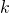andbe two multiplicatively independent positive integers and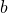be an integer with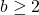. Let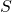be a finite set of integers. Nishioka proved that for any algebraic number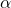with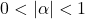the infinite products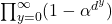(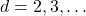) are algebraically independent over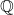. As her result, for example, the transcendence of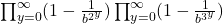is deduced. On the other hand, Tachiya, Amou–Väänänen investigated the certain infinite products which satisfy infinite chains of Mahler functional equation. The special case of the result of Tachiya shows that the infinite product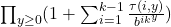with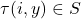(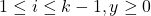) is either rational or transcendental.

In this paper, we prove that the infinite product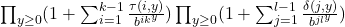with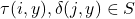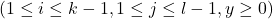is either rational or transcendental. Moreover, we give sufficient conditions thatis transcendental.

A primality test for Kpn + 1 numbers and a generalization of Safe primes and Sophie Germain primes
Original research paper. Pages 62—77
Abdelrahman Ramzy

In this paper, we provide a generalization of Proth’s theorem for integers of the form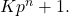In particular, a primality test that requires a modular exponentiation (with a proper base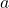) similar to that of Fermat’s test without the computation of any GCD’s. We also provide two tests to increase the chances of proving the primality of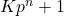primes. As corollaries, we provide three families of integers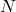whose primality can be certified only by proving that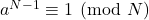(Fermat’s test). One of these families is identical to Safe primes (since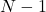for these integers has large prime factor the same as Safe primes). Therefore, we considered them as a generalization of Safe primes and defined them as-Safe primes. We address some questions regarding the distribution of those numbers and provide a conjecture about the distribution of their generative numbers-Sophie Germain primes which seems to be true even if we are dealing with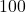,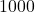, or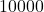digits primes.

Sums involving the binomial coefficients, Bernoulli numbers of the second kind and harmonic numbers
Original research paper. Pages 78—97
Necdet Batır and Anthony Sofo

We offer a number of various finite and infinite sum identities involving the binomial coefficients, Bernoulli numbers of the second kind and harmonic numbers. For example, among many others, we prove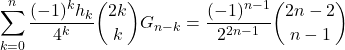and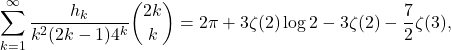where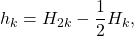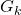are Bernoulli numbers of the second kind, and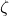is the Riemann zeta function. We also give an alternate proof of the series representations for the constants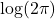and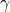given by Blagouchine and Coppo.

A generalized computation procedure for Ramanujan-type identities and cubic Shevelev sum
Original research paper. Pages 98—129
Peter J.-S. Shiue, Anthony G. Shannon, Shen C. Huang, Jorge E. Reyes

A generalized Computation procedure for construction of the Ramanujan-type from a given general cubic equation and a cosine Ramanujan-type identity is developed from detailed analyses of the properties of Ramanujan-type cubic equations. Examples are provided together with cubic Shevelev sums.

On certain equations and inequalities involving the arithmetical functions φ(n) and d(n) – II
Original research paper. Pages 130—136
József Sándor

In papers  and  we have studied certain equations and inequalities involving the arithmetical functions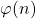and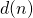. In this paper we will consider some other equations. Some open problems will be stated, too.

Congruences for harmonic sums
Original research paper. Pages 137—146
Yining Yang and Peng Yang

Zhao found a curious congruence modulo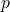on harmonic sums. Xia and Cai generalized his congruence to a supercongruence modulo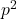. In this paper, we improve the harmonic sums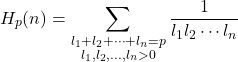to supercongruences modulo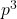and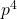for odd and even where prime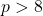and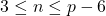.

A note on edge irregularity strength of firefly graph
Original research paper. Pages 147—153
Umme Salma, H. M. Nagesh and D. Prahlad

Let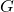be a simple graph. A vertex labeling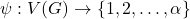is called-labeling. For an edge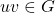, the weight of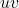, written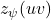, is the sum of the labels ofand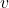, i.e.,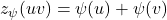. A vertex-labeling is said to be an edge irregular-labeling ofif for every two distinct edgesand,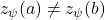. The minimumfor which the graphcontains an edge irregular-labeling is known as the edge irregularity strength ofand is denoted by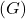. In this paper, we find the exact value of edge irregularity strength of different cases of firefly graph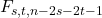for any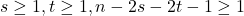.

Hybrid hyper-Fibonacci and hyper-Lucas numbers
Original research paper. Pages 154—170
Yasemin Alp

Different number systems have been studied lately. Recently, many researchers have considered the hybrid numbers which are generalization of the complex, hyperbolic and dual number systems. In this paper, we define the hybrid hyper-Fibonacci and hyper-Lucas numbers. Furthermore, we obtain some algebraic properties of these numbers such as the recurrence relations, the generating functions, the Binet’s formulas, the summation formulas, the Catalan’s identity, the Cassini’s identity and the d’Ocagne’s identity.

Objects generated by an arbitrary natural number. Part 3: Standard modal-topological aspect
Original research paper. Pages 171—180
Krassimir Atanassov

The set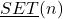generated by an arbitrary natural number, was defined in . There, and in , some arithmetic functions and arithmetic operators of a modal type are defined over the elements of. Here, over the elements ofarithmetic operators of a topological type are defined and some of their basic properties are studied. Perspectives for future research are discussed.

Corrigendum to: “Some modular considerations regarding odd perfect numbers – Part II” [Notes on Number Theory and Discrete Mathematics, 2020, Vol. 26, No. 3, 8–24]
Corrigendum. Pages 181—184
Jose Arnaldo Bebita Dris and Immanuel Tobias San Diego